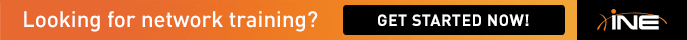# task 4.4 (ip ospf cost)

hello all,

why in the solution guide for lab3, you have adjusted the cost (ip ospf cost 65534) when dealing with virtual-circuit. I have got the same output without this command? is it correct??

Thanks
Lamine

• The cost is required because the serial link between R4 and R5 is a backup link, and should only be used when R4's FR connection to R5 is down. (as per the Q)
• hi mate

By ne chance on lab 3 do you know how they calculated the cost on task 4.6
it says cost = reference bw / interface bw

can you calculate the following

BW OSPF COST
10,000 2
10 2000
1,544 12953

If you can write out the formult in full for the 1st one wil be appreciated

thanks
• 1: 10,000

cost = reference bw / interface bw
by default reference b/w is 100mbs meaning 100*10^3*10^3=100000000 bits.